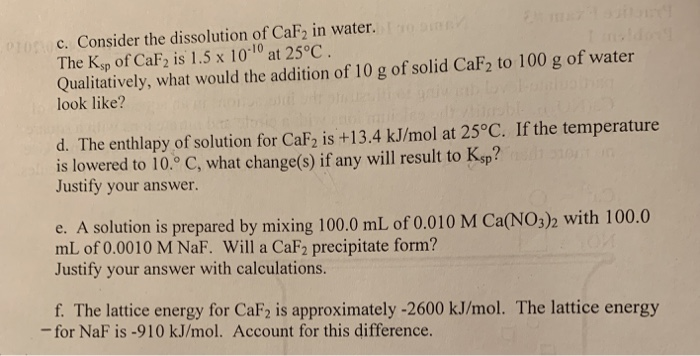Homework Help Question & Answers

# C. Consider the dissolution of CaF2 in water. The Ksp of CaF2 is 1.5 x 1010 at 25°C Qualitatively...c. Consider the dissolution of CaF2 in water. The Ksp of CaF2 is 1.5 x 1010 at 25°C Qualitatively, what would the addition of 10 g of solid CaF2 to 100 g of water look like? d. The enthlapy of solution for CaF2 is +13.4 kJ/mol at 25°C. If the temperature is lowered to 10° C, what change(s) if any will result to Ksp? Justify your answer. e. A solution is prepared mL of 0.0010 M NaF. Will a CaF2 precipitate form? Justify your answer with calculations. red by mixing 1000 ml. oro.oio M CaNOs)h with 100.0 f. The lattice energy for CaF2 is approximately -2600 kJ/mol. The lattice energy -for NaF is -910 kJ/mol. Account for this difference.

c. Density of water=1 g/mL

So volume of 100 g water=mass/density=100 g/1 g/mL=100 mL=100 mL/1000 mL/L=0.1 L (1000 mL=1 L)

Molar mass of CaF2=Molar mass of Ca+2xmolar mass of F=40 g/mol+2x19 g/mol=40 g/mol+38 g/mol=78 g/mol

Number of moles of CaF2=mass/molar mass=10 g/78 g/mol=0.13 mol

Molarity of CaF2=number of moles/volume of solution (L)

=0.13 mol/0.1 L=0.013 M

CaF2 dissociates as shown in the solution0.013 M 2x0.013 M

=0.026 M

Reaction quotient Q==0.013 M x (0.026 M)2=8.8x10-6 M3

As Q>Ksp so CaF2 will precipitate out. Qualitatively, 10 g Calcium fluoride added to 100 g water doesn't dissolve completely.

d. Since enthalpy of dissolution is positive, it means it is an endothermic reaction. On lowering the temperature of an endothermic reaction it moves in a direction so as to increase the temperature i.e. in backward direction. So lowering the temperature reduces the solubility of calcium fluoride and hence lowers its Ksp.

e. Number of moles of calcium nitrate=Molarity x Volume

=0.010 M x 100 mL/1000 mL/L

=0.001 mol

Concentration of calcium nitrate in diluted solution=number of moles/volume of the solution=0.001 mol/200 mL/1000 mL/L=0.005 M

1 molecule of CaF2 gives 1 Ca+2 in solution

So concentration of Ca+2 in solution=0.005 M

Number of moles of sodium fluoride=Molarity x Volume

=0.0010 M x 100 mL/1000 mL/L

=0.0001 mol

Concentration of NaF in diluted solution=number of moles/Volume (L)

=0.0001 mol/200 mL/1000 mL/L=0.0005 M

1 molecule of NaF gives 1 F- in solution

So concentration of F- in diluted solution=0.0005 M

Reaction quotient Q==0.005 M x (0.0005 M)2=1.25x10-9 M3

As Q>Ksp, a precipitate of calcium fluoride will form.

f. In CaF2, we have ionic bonding between Ca+2 (bivalent cation) and F-​​​. In NaF the ionic bonding is between Na+ (monovalent cation) and F-. So the electrostatic forces of attraction is more in CaF2 than in NaF due to higher charge on calcium ion than sodium ion. So lattice energy is higher for calcium fluoride than NaF.

##### Add Answer of: C. Consider the dissolution of CaF2 in water. The Ksp of CaF2 is 1.5 x 1010 at 25°C Qualitatively...
More Homework Help Questions Additional questions in this topic.

• #### Qualitatively describe the equilibrium position of a boom, and reaction forces of a boom in...

Need Online Homework Help?

Get FREE EXPERT Answers
WITHIN MINUTES
Related Questions